# Angles Of Elevation And Depression Study Of Measurement Using Clinometers

Welcome to Class !!

In today’s Mathematics class, We will be talking about Angles of Elevation and Depression. We hope you enjoy the class!CONTENT:

i)   Horizontal and vertical lines

ii) Angles of elevation

iii) Measuring angles of elevation and depression.

### Horizontal and Vertical Lines

Horizontal lines are lines that are parallel to the surface of the earth. For example, the surface of a liquid in a container, the floor of a classroom, etc. See the diagram below: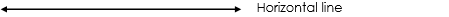Vertical lines are lines that are perpendicular to the horizontal surface, e.g. wall of a classroom, a swing pendulum, etc.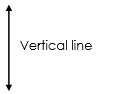Evaluation:

Say whether the following are horizontal or vertical or neither.

1. a) A tabletop  b) A door       c) A table leg           d) Top edge of a wall.

NGM Bk 2 Chapter 20, Page 165

Essential Mathematics for JSS Bk 2, Chapter 17, Pg 173

### Angles of Elevation

The angle of elevation of an object for a given point above the surface of the earth is the angle formed between the horizontal plane and the viewpoint of the object. See the diagram below.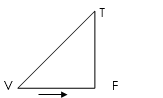Horizontal plane

V = Viewpoint,     T = Top where the object is,       F = Foot of the vertical plane

e =  angle of elevation

Reference:

NGM Bk 2 Chapter 20, Page 165

Essential Mathematics for JSS Bk 2, Chapter 17, Pg 173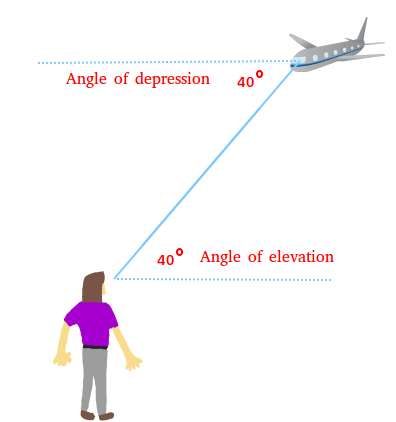### Angle of Depression

The angle of depression of an object from a given point T is the angle from the horizontal line above the earth’s surface and the vertical surface.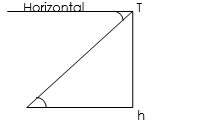Thus, the angle of elevation is equal in size to the angle of depression. (alternate angles are equal i.e. d = e)

Measuring Angles of Elevation and Depression

When constructing angles of elevation and depression, the use of scale drawings is necessary in order to have effective construction of angles

Worked Examples

1. Consider the diagram below; find the height of the flagpole to the nearest metre using a suitable scale.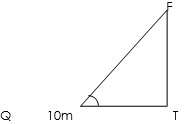Solution

By construction, choose a scale of 1cm to represent 2m.

The height of the flagpole PT = 3cm converted to m, will give 2 × 3 = 6.

Example 2: The angle of elevation of the top of a tower to a point 42m away from its base on level ground is 360, find the height of the tower.

Solution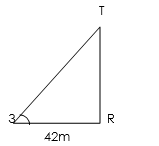By construction, using a suitable scale of 1cm represented by 6m, then PR = 42/6 = 7cm.

The length TR = 5.0cm. Converting back to metre, we have;

Length TR = 5 × 6 = 30m

Example 3: From the top of a building 20m high, the angle of depression of a car is 450, find the distance of the car from the foot of the building.

Solution

Rough sketch: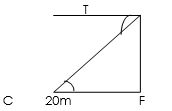T = Top of the building, C = Car, F = Foot of the building

CF is the distance of the car from the foot of the building.

Since the angle of depression equals the angle of elevation;

By construction, using a suitable scale of 1cm represents 5m

For 20m, we have 20/5 = 4cm

Length CF = 4cm

By conversion, length CF = 4 × 5 = 20m.

Evaluation:

1. A tower PQ is 10m high, if the distance from point R to P is 50m on the ground, find the angle of elevation of Q from R.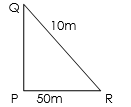1. From the top of a cliff of 200m high, Dele observes that the angle of depression of a boat at sea is 350, find the distance between the boat and the foot of the cliff.

NGM Bk 2 Chapter 20, Page 166 – 169

Essential Mathematics for JSS Bk 2, Chapter 17, Pg 176 – 177.

WEEKEND ASSIGNMENT

Objective

1. Calculate the size of the fourth angle if three angles of a quadrilateral are 650, 1150, and 1250 respectively.   a) 350      b) 550      c) 450       d) 750
2. Calculate the number of sides of a regular polygon whose total angle is 10800.    a) 4       b) 6           c) 8           d) 10
3. PQRS is a rectangle with sides 3cm and 4cm, if its diagonal cross at O, calculate the length of PO.               a) 3.5cm   b) 5.0cm     c) 2.5cm      d) 4.0cm
4. If the angles of a pentagon could be x, 2x, 4x and 5x respectively, what would be the value of x? a) 600 b) 900 c) 150      d) 300

THEORY

1. From the top of a building 50m high, the angle of depression of a car is 550, find the distance of the car from the foot of the building.
2. Find the height of the flagpole in the diagram below to the nearest metre.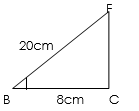We have come to the end of this class. We do hope you enjoyed the class?

Should you have any further question, feel free to ask in the comment section below and trust us to respond as soon as possible.

In our next class, we will be talking about Bearing. We are very much eager to meet you there.

Are you a Parent? Share your quick opinion and win free 2-month Premium Subscription

Don`t copy text!